# QuasiTiler 3.0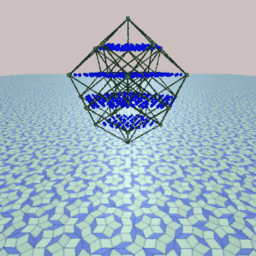## by Eugenio Durand

World Wide Web front end by Paul Burchard, Daeron Meyer and Eugenio Durand at the Geometry Center, University of Minnesota, March 1994.

# Introduction

QuasiTiler draws Penrose tilings and their generalizations. This document explains the interesting geometry involved in the processes. The concepts involved are surprisingly simple. The only apparent hurdle that we have to overcome is working in spaces with more than 3 dimensions. However in the next section we start with examples in 2 and 3 dimensions where our intuition is useful. Then we extend the same concepts to more dimensions. I hope that you get some insight on the geometry of higher-dimensional spaces by reading this document and by experimenting with QuasiTiler.

Penrose tilings are well known because of their interesting and sometimes intriguing properties; for example, they are locally but not globally symmetric under 5-fold rotations, quasi-periodic with respect to translations, self-similar, and more. See the references at the end of this document if you want to find more about Penrose tilings. If you do not know what a Penrose tiling is, there is one in the background of the image at the top of this document.

N.G.deBruijn in 1981 showed that Penrose tilings can be seen as the projection of a relatively simple object in 5-dimensional space. QuasiTiler uses deBruijn's approach to generate tilings.

The original version of QuasiTiler is a NeXTSTEP application, with more features than the ones possible to implement over the web. It is available by anonymous ftp from this server. See the reference at the end of this document.

# Geometry of Quasicrystals

## One dimension, 2 dimensions, n dimensions

Before we jump into 5 dimensions, let's start with 2. We look first at the coordinate plane, which is a 2-dimensional space. In this plane we consider the points with integer coordinates. We call these points an integer lattice. The lattice together with the corresponding edges fills the plane with a grid of squares. We pick any line in the plane and we call it line E for convenience.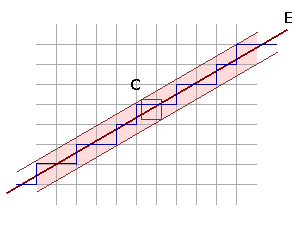We look now to a region of the plane around the line E. This region is just wide enough to contain any square with side length 1 centered at any point on the line E. We call this region C. Now we select all the points in the lattice that happen to be inside the region C. We also select all the edges that join these selected points. We obtain a funny looking staircase curve as result. Now we project the staircase perpendicularly onto E. Adjacent edges of the staircase collapse to adjacent line segments in E. We have as result a tiling of this one-dimensional space.

Observe that we can choose the line E anyway we want, obtaining a different staircase each time. An important property depends on the slope of E: if we choose the slope of E to be a rational number then the pattern of the staircase and the projected tiling repeats itself periodically; but if the slope is an irrational number the pattern is not periodic. However in this last case, if we choose any piece of the staircase (of finite size) the chosen piece will be repeated an infinite number of times at different places all over the region C: we get a quasiperiodic staircase.

QuasiTiler does exactly the same thing. But instead of using the line E and a square grid on the plane, it uses a plane and an integer lattice in spaces of dimension 3, 4, 5 or more. By passing a higher-dimensional cube along E, we get a 2-dimensional staircase siting in a higher-dimensional space. QuasiTiler then projects the staircase surface onto the plane E. The result is the tiling that you see.

Let's work with the idea in 3 dimensions first: instead of a grid of squares in the plane we consider a 3-dimensional grid of cubes, and a 2-dimensional plane crossing them instead of a line. The vertices of the cubes are the points on the integer lattice. We take a plane E, and as before slice the space with it and look at the region C around the plane E. This region is just wide enough to contain all the cubes of side-length 1 centered at any point in the plane E. We select then all the lattice points inside this region C, together with the corresponding edges and faces. The result is an arrangement of steps, which we project into the plane E. You can see in the next image two different results obtained with QuasiTiler by choosing two different planes.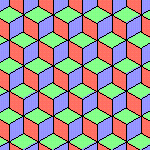With QuasiTiler we can browse in 4, 5 or more dimensions by taking 2-dimensional "slices" of the corresponding lattice. The results will get more interesting. The more dimensions we have the more things can happen. For example, Penrose tilings are just a particular class of these slices in 5-dimensional space.

QuasiTiler shows also a different view of the same staircase surface sitting in n-dimensional space by projecting it into the space orthogonal (= perpendicular) to the plane E. The lattice points in C all project into the interior of the projection of the n-dimensional cube. The image below corresponds to a Penrose tiling.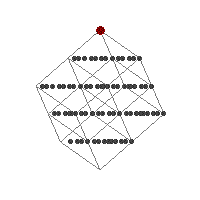The gray points correspond to the lattice points near the plane E. The region C is represented by the light gray lines, which are the edges of an n-dimensional cube of side-length 1, with n equal to 5 in this particular case. Finally the large red dot represents the origin of the coordinate system, and indicates the position of the plane E and the region C relative to the integer lattice and the ambient space. You are in control of this red dot in the program.

Notice that the orthogonal space to E has dimension n-2, but QuasiTiler shows you only a 2-dimensional view since a computer screen is flat. This is good enough in most cases anyway. However there is room to improve this flat view. For example, the image at the top of this document shows a 3-dimensional view in perspective of the same object represented in the image above.

## Doing it by numbers

So far we have talked about an arbitrary plane in n-dimensional space, but we haven't said how we are going to manipulate such an object. We will use some linear algebra from here to the end of this section (do not worry if you do not understand all the details -- the geometry explained up to this point is the important part). We are just going to attach some numbers to the geometry so we can conveniently deal with it. QuasiTiler keeps track of the numbers, but also allows you to deal directly with the numbers if you want.

The plane E is specified by one position vector and two direction vectors. In QuasiTiler, we call the position vector the offset, and the two direction vectors the generators. There are some conditions that we are going to impose on these vectors. The first condition is that the two generators need to be linearly independent; this is a necessary condition, otherwise we would get a line instead of a plane. The next condition is that that the generators are orthogonal to each other and of length 1; this is not necessary, but it will be helpful, as we will see later. The final condition is that offset has to be orthogonal to the plane E; this condition is just to simplify the computations. QuasiTiler allows you to enter the numbers directly, and makes sure that all the conditions are satisfied.

To ensure that the offset is orthogonal to the plane E, the offset vector is always represented as a vector in the orthogonal complement of the plane E, which is an (n-2)-dimensional space. The position of the large red dot in QuasiTiler's offset control is determined by the first two coordinates of the offset.

Let's see now the advantages of having the generators orthogonal to each other and of length 1. We consider the canonical basis of the ambient space. The canonical basis is just the vectors of the form

```     ( 1, 0, 0, . . . ),
( 0, 1, 0, . . . ),
( 0, 0, 1, . . . ), . . .
```
When the condition on the generators is satisfied, we can tell right away the coordinates of the projection onto the plane E of the k-th canonical basis vector; these coordinates are the same as the k-th coordinates of the two generators. And these projections of the canonical basis are important for us, since they are precisely the edges of our tiles, as we explain next.

Remember that the edges of the surface in the higher dimensional space are just the edge of some n-dimensional cube with integer coordinates vertices. And the edges of any of these n-dimensional cubes are a translation of one of the vectors in the canonical basis, which are then projected onto the plane E. Thus all the edges in the tiling are just the projection of one of the vectors from the canonical basis translated to the corresponding vertex. Furthermore any tile is just a parallelogram determined by a pair of these edges. In other words, the projections of the canonical basis give the coordinates to build the tiles.

## What is the picture at the top of the document?

The picture illustrates the geometry behind QuasiTiler. A tiling obtained by QuasiTiler comes from the projection of a particular surface in n-dimensional space determined by a set of points with integer coordinates in n-dimensional space. You can see on the picture two different projections of the same surface.
• In the background is a Penrose tiling, which is the projection of the surface onto the generating plane E (see the previous section).
• In the foreground is the projection of the vertices of the surface onto the space orthogonal to the generating plane. The vertices of the surface are shown as blue dots. Also shown in green are the edges and vertices of the projection of a 5-dimensional unit "cube."
Notice the vertices of the surface also have structure when projected in the orthogonal space to the plane. For example in this particular case the points are confined to 4 planes. In fact the properties of the Penrose tiling are encoded in the orthogonal view. The position of each projected vertex inside the projected 5-dimensional cube determines the configuration of tiles at the corresponding point in the tiling.

## Quasicrystals

Perhaps you are wondering what might be the results of taking a 3-dimensional slice instead of a 2-dimensional one. It would be a structure similar to the Penrose tilings, whose building units fill space quasiperiodically. Although for nearly 200 years this was assumed to be impossible, such crystals really exist! See the references at the end of this document.

# Using QuasiTiler

QuasiTiler is implemented as a HTML fill-out form. It is divided in two areas: the first is the tile display together with the input widgets to control how the tiling is displayed. The second area has the widgets to control the generating plane.

## Tile Display

The tiling computed by QuasiTiler is the top-most image. You can control this display in several ways. You can choose which rectangular region of the tiling is displayed, and adjust some aspects of how the tiles are drawn. You have the following options:
• To display a different region of the tiling, select any point in the image. A new image will be computed with the selected point at the the center.
• To change the size of the displayed region you can choose one of the following zoom options:
• None. The new region of the tiling has the same size as the last one; this is the default.
• Out. The new region will be larger, with more and smaller tiles.
• In. The new region will be smaller, with fewer and bigger tiles.
• To change how the tiles are drawn, you can choose any of the followingdisplay options:
• Edges. If selected, the edges will be displayed as black lines; no edges are displayed otherwise.
• Tiles. If selected the tiles are shaded with a color depending the shape of the tile; the tiles are not displayed otherwise.
• Color. Determines whether the tiles are painted with different colors or with shades of gray. Keep in mind that the colors have no particular meaning, they are chosen just to underline the symmetry of the tiling.
• GIF Only / PostScript Only. Select one of these if you would like to have the next computed image of the tiling returned to you as a stand alone image document. Otherwise QuasiTiler will return a new HTML form with the new tiling included as an inlined image to use interactively.

## Generating Plane

In the the two dimensional example above, we can determine the line E by choosing a point and a direction. A convenient way to describe these point and direction is with two vectors. In the same way a plane can be determined by three vectors. One vector determines a point through which the plane goes; we call this the offset. The other two determine the direction of the plane; we call these the generators.

### Changing the Offset

The program allows you to modify this parameter in two different ways. One is to select a point in the image that looks similar to the following one.In this image you can see the relative positions of the lattice points inside the region R. The view is a projection into two of the orthogonal directions to the generating plane. You are in control of the red dot, which represents the center of the coordinate system, the origin. Whenever you select a point in this image the origin will move there; the offset of the plane E will change correspondingly. Keep in mind that this method you can only change two coordinates of the offset vector, however this gives more than enough room to explore.

If you insist on changing the offset in all its coordinates you can use the second method. At the bottom of the form there are three columns of numbers. The coordinates of the offset vector are on the first column. Type any values you want and submit the form when you are done. On the technical side, this vector is expressed in the coordinates of the space orthogonal to the generating plane.

### Changing the Generators

QuasiTiler presents you with a sample of the current tiles in an image similar to the one below. The "direction" of the plane, which is given by the generators, determines the shape of the tiles. You can change the generators by selecting a point in the image.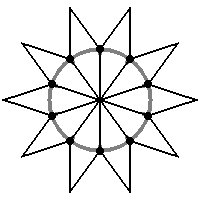The fat points in the image represent the projection of the canonical basis of the ambient space into the generating plane. When you select a point in this image, the program will move the generating plane so that the nearest fat point will move as close as possible to your selection, dragging with it the shape of the tiles. Some directions and shapes will give more pleasing tilings than others. The default values produce tiles symmetric under rotations.

As with the offset, it is possible for you to change the coordinates of generators directly. The coordinates of each generator are on the two columns at the end of the form. Type any values you want and submit the form when you are done. Notice that there are some conditions that must be satisfied by these numbers; QuasiTiler modifies your input to enforce those conditions. This is explained in a previous section.

## Things to do with QuasiTiler

Try any of the display options.
You will always get an interesting result.
Select a point in the offset image.
You will get a different tiling using the same tiles. Notice how the tiling changes with as you change the relative positions of the plane and the lattice. This is the favorite control of the author. You will always get an interesting result.
Change the dimension of the ambient space.
Start with 3 dimensions; the image are not as interesting but you will recognize the cubes that project as the tiling. As you increase the dimension to 4 or 5, try to keep the same image of "stacks of cubes."
Redecorate the bathroom :-)
The tiles are always parallelograms. You can read the shape of each from the numbers in the bottom of the form. Check the section explains these numbers. But in the default symmetrical case is easier: all the edges have the same length, and the angles are multiples of (180 degrees) / (dimension of ambient space). You have to let me know if you actually get involved in a project like this!

## Things that can go wrong

Time out error
The server has limit on the time it can spend to satisfy your request. Possible reasons for going over the limit are: you zoom out too much, you selected a large dimension, the server is working on other requests simultaneously to yours, or a combination of any of the previous. Try to zoom in or select a smaller dimension.
Tiles with strange shapes
Some choices of generators produce very asymmetrical tiling. Start over or change dimension to get the default symmetrical settings.
Missing tiles
In some rare occasions a point in the integer lattice is to close to the border of the region determining the tiling. QuasiTiler can not tell if the point is inside or outside in such case. To be on the safe side, QuasiTiler guess is that the point is outside. But sometimes the guess is wrong and there is a hole in the tiling. A solution is to change some of the less significant digits in the offset.
The demo is not working
Chances are that your WWW browser does not support forms and/or inlined images. Try to get a newer version that supports this features.

# Conclusion

I hope that you have fun and learn with QuasiTiler, as I did writing it. I just would like to make the following point. There is somehow a general idea that 4, 5 or more dimensions have some sort of mystical properties. I find this idea surprising, maybe even disturbing: as you can see in this program, n-dimensional spaces are just mathematical concepts, interesting to study on their own, and also useful as tools to find solutions to other problems. For example QuasiTiler uses them to produce interesting and nice looking patterns.

## Acknowledgements

The original motivator to write QuasiTiler was Prof. Marjorie Senechal, Dept. of Mathematics, Smith College, Northampton, MA. During her visit to the Geometry Center she required a convenient way to generate Penrose tilings and so the project started. She has provided the expertise on the subject essential to write QuasiTiler and this document.

# References

## Penrose tilings

Martin Gardner, Mathematical Recreations, Scientific American, 236 (January 1977) 110-121 . This is a very enjoyable article.

Martin Gardner, Penrose Tiles to Trapdoor Ciphers, W.H.Freeman, New York, 1989.

Roger Penrose, The Emperor's New Mind, Oxford University Press, New York, 1989. Yes, he is the same Penrose as in "Penrose tiles." The tiles are mention only briefly though.

N.G.deBruijn, Algebraic theory of Penrose's nonperiodic tilings of the plane, I, II, Nederl. Akad. Wetensch. Indag. Math. 43 (1981) 39-52, 53-66. This is deBruijn original paper, hard to read.

Stan Wagon, Mathematica in Action, W.H.Freeman, New York, 1991, chapter 4. Here you can find how to generate Penrose tilings using the Mathematica software, together with a nice explanation of some of their properties. The approach used here is different from QuasiTiler. It has further references too.

Ivars Peterson, The Mathematical Tourist: Snapshots of Modern Mathematics, chapter 7, W.H.Freeman, New York, 1988. Here the book's author stops short of exploring the same ideas as in this document.

## Quasicrystals

Marjorie Senechal, online article on this server.

Marjorie Senechal, Quasicrystals and Geometry, Cambridge University Press, 1995. To appear.

D. R. Nelson, Quasicrystals, Scientific American, 255 (August 1986) 42-51.

D. R. Nelson and B. I. Halperin, Pentagonal and icosahedral order in rapidly cooled metals, Science, 229 (19 July 1985) 233-238.

## QuasiTiler

On the Geometry Forum on this server, there is an article regarding QuasiTiler with a brief explanation.

Check also a another explanation to the mathematics behind QuasiTiler, by Prof. Marjorie Senechal, an expert on the subject.

QuasiTiler is also available as a NeXTSTEP application by anonymous ftp from the Geometry Center FTP. To download the Multi-Architecture Binary (Motorola, Intel, HP PA-RISC) /pub/software/QuasiTiler-3.0.tar.Z click here.

## Other Resources

Many other references and software packages related to tilings are detailed in Doris Schattschneider's list of tesselation resources.

Any comments and suggestions concerning this web server are welcome and should be e-mailed to webmaster@geom.umn.edu.```The Geometry Center
University of Minnesota
400 Lind Hall
207 Church Street S.E.
Minneapolis, MN  55455

```